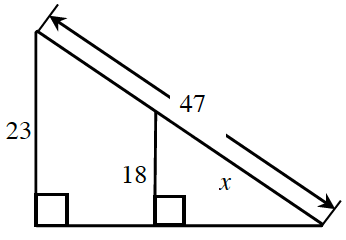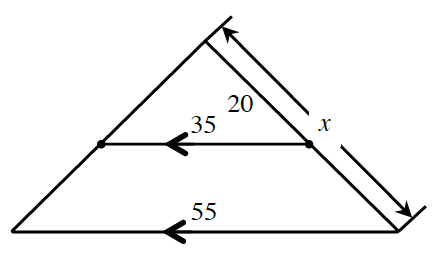### Home > CCA2 > Chapter 5 > Lesson 5.2.1 > Problem5-64

5-64.

Solve for $x$ in the following diagrams.

1.The triangles are similar. Recall that in similar triangles corresponding sides are proportional.

$\frac{18}{x} = \frac{23}{47}$

1.See part (a). Try drawing two separate triangles.

$x \approx 31.43$